Case Based Questions (MCQ)

Chapter 9 Class 12 Differential Equations
Serial order wise

## A Veterinary doctor was examining a sick cat brought by a pet lover. When it was brought to the hospital, it was already dead. The pet lover wanted to find its time of death. He took the temperature of the cat at 11.30 pm which was 94.6° F. He took the temperature again after one hour; the temperature was lower than the first observation. It was 93.4° F. The room in which the cat was put is always at 70° F. The normal temperature of the cat is taken as 98.6° F when it was alive. The doctor estimated the time of death using Newton law of cooling which is governed by the differential equation: dT/dt∝ (T – 70), where 70° F is the room temperature and T is the temperature of the object at time t. Substituting the two different observations of T and t made, in the solution of the differential equation dT/dt = 𝑘(𝑇 − 70) where k is a constant of proportion, time of death is calculated.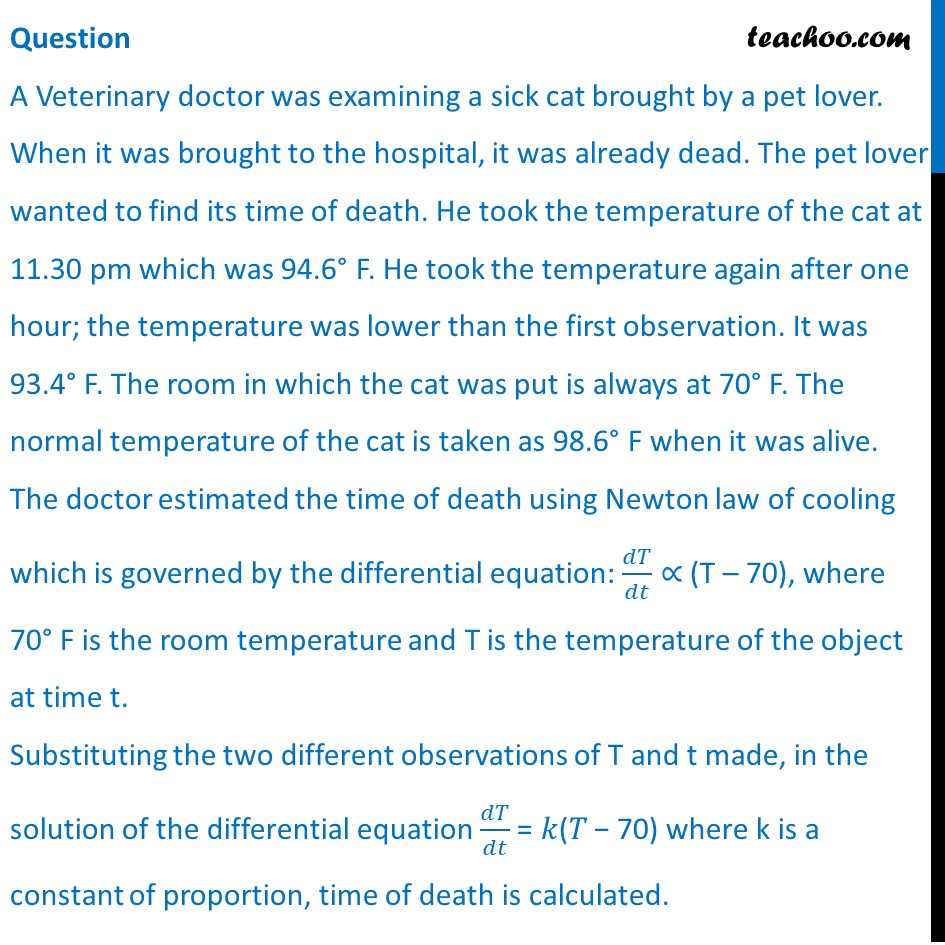## State the degree of the above given differential equation.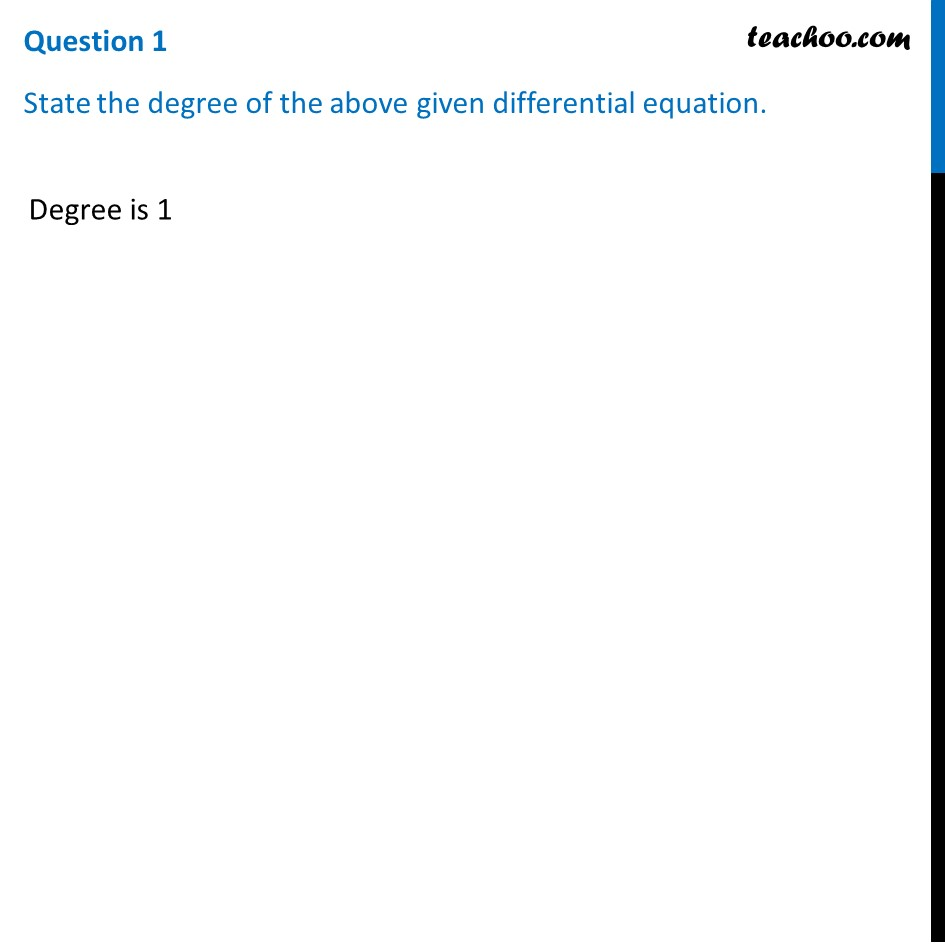## (d) all of the above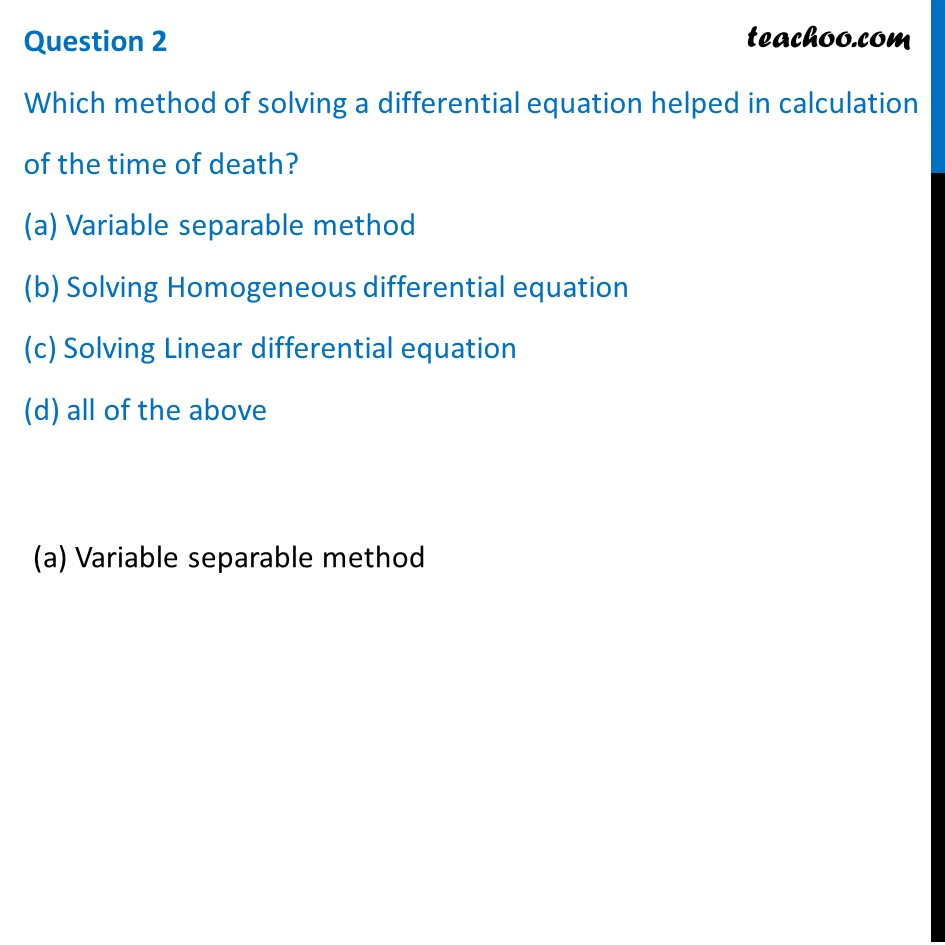## change? (Yes/No)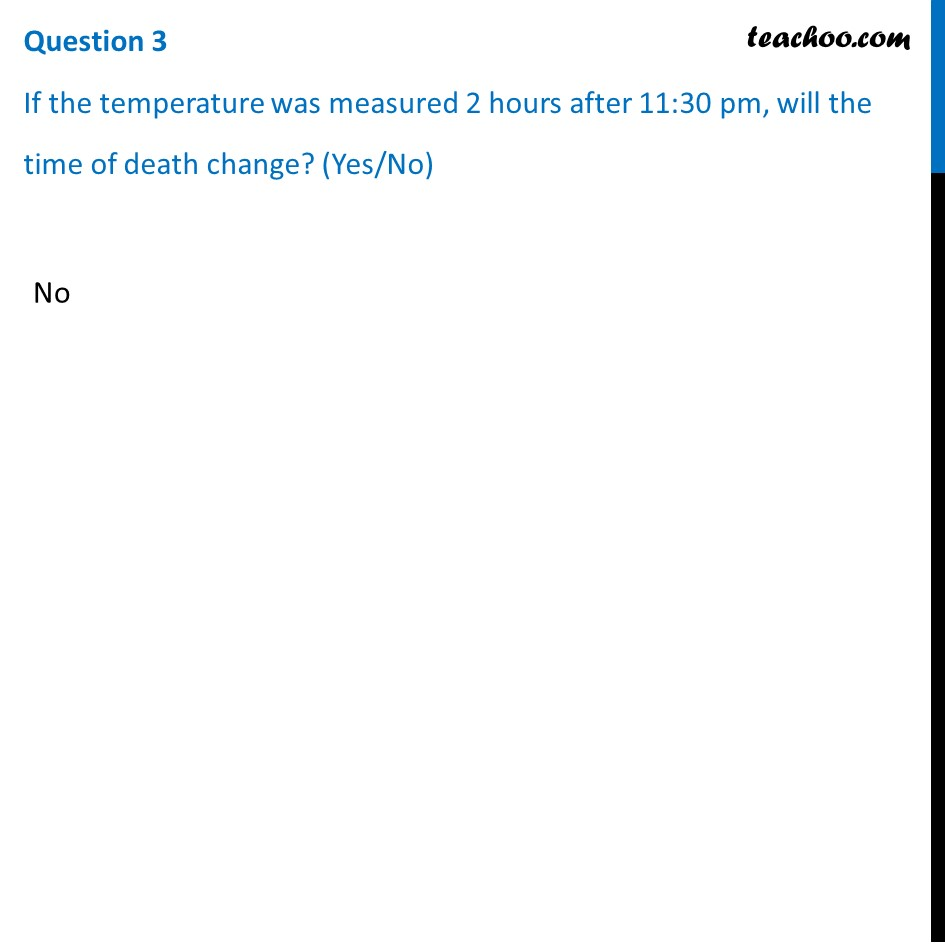## The solution of the differential equation dT/dt = 𝑘(𝑇 − 70) is given by, (a) log⁡|T-70| =kt +C (b) log⁡ |T-70| =log⁡ |kt|+C (c) T-70 = kt  +C (d) T-70 = kt C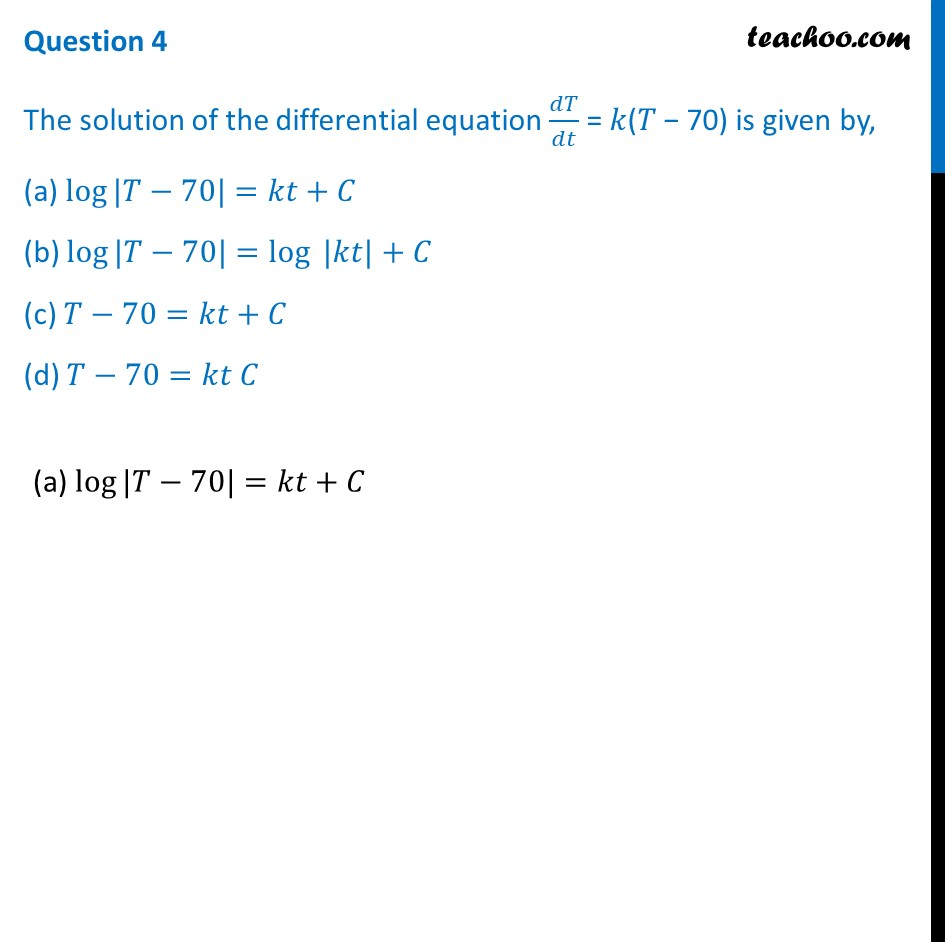## (d) log 2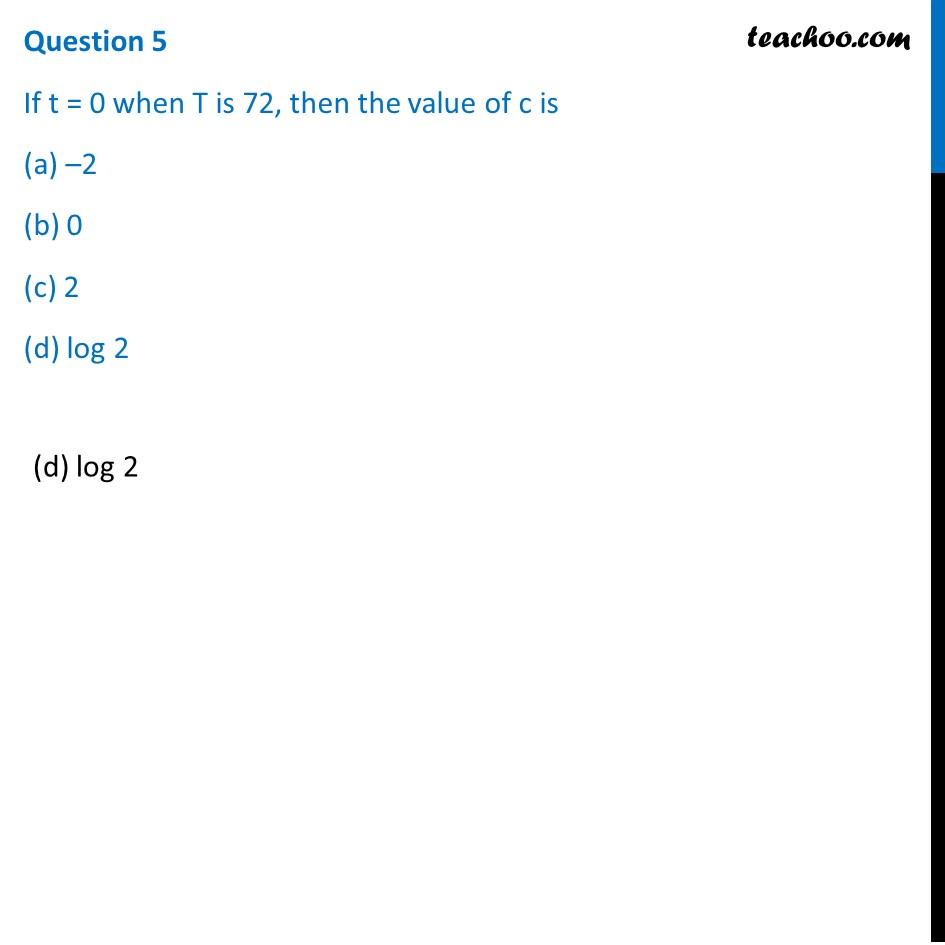Learn in your speed, with individual attention - Teachoo Maths 1-on-1 Class

### Transcript

Question A Veterinary doctor was examining a sick cat brought by a pet lover. When it was brought to the hospital, it was already dead. The pet lover wanted to find its time of death. He took the temperature of the cat at 11.30 pm which was 94.6° F. He took the temperature again after one hour; the temperature was lower than the first observation. It was 93.4° F. The room in which the cat was put is always at 70° F. The normal temperature of the cat is taken as 98.6° F when it was alive. The doctor estimated the time of death using Newton law of cooling which is governed by the differential equation: 𝑑𝑇/𝑑𝑡∝ (T – 70), where 70° F is the room temperature and T is the temperature of the object at time t. Substituting the two different observations of T and t made, in the solution of the differential equation 𝑑𝑇/𝑑𝑡 = 𝑘(𝑇 − 70) where k is a constant of proportion, time of death is calculated.Question 1 State the degree of the above given differential equation.Degree is 1 Question 2 Which method of solving a differential equation helped in calculation of the time of death? (a) Variable separable method (b) Solving Homogeneous differential equation (c) Solving Linear differential equation (d) all of the above(a) Variable separable method Question 3 If the temperature was measured 2 hours after 11:30 pm, will the time of death change? (Yes/No)No Question 4 The solution of the differential equation 𝑑𝑇/𝑑𝑡 = 𝑘(𝑇 − 70) is given by, (a) log⁡〖|𝑇−70|〗=𝑘𝑡+𝐶 (b) log⁡〖|𝑇−70|〗=log⁡〖 |𝑘𝑡|+𝐶〗 (c) 𝑇−70=𝑘𝑡+𝐶 (d) 𝑇−70=𝑘𝑡 𝐶(a) log⁡〖|𝑇−70|〗=𝑘𝑡+𝐶 Question 5 If t = 0 when T is 72, then the value of c is (a) –2 (b) 0 (c) 2 (d) log 2(d) log 2